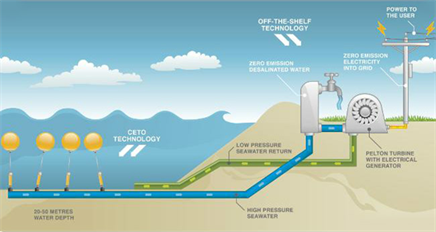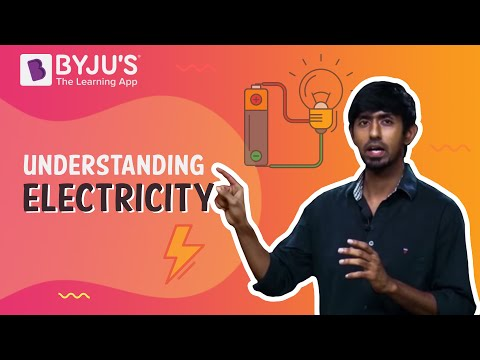# Wave Power

## What is Wave Power?

You may have noticed that there is a certain kind of air force when you go near any seashore. This is due to a type of energy that gets transport by wind waves. This energy is also known as wave power. We can define it as:

Wave power is the capture of energy of wind waves to do useful work

Example:

• Water Desalination
• Electricity Generation
• Pumping Water

When the wind passes on the water surface, it leads to the pressure difference between the upper and bottom wind which results in the generation of waves. This wave energy which is captured can be used for several useful works namely water distillation, electric generator, and WEC.

The motion of waves contains a different degree of energy. The electromagnetic waves from the wave energy give us energy for sustaining life on earth. Water desalination or the pumping of water into reservoirs are some uses of the wave power, electricity generation, etc. Some main properties of waves include speed, frequency, period, amplitude, and wavelength.### Ocean wave energy and its types

Ocean wave energy is a natural source of energy that is directly captured from the pressure fluctuations below the surface or from the surface area itself. They are therefore of two types namely:

• Ocean Thermal Energy
• Ocean Mechanical Energy

In Ocean Mechanical Energy, electricity is produced by taking energy from the ocean in three main types:

1. The channel systems, which are used to funnel the waves into reservoirs.
2. Float systems are used to run the hydraulic pumps.
3. The column system is used to compress the air present within the containers.

Ocean wave power is then used to rotate the turbine or the generator and the electricity is produced.

Ocean Thermal Energy uses the temperature of the surface sea waters to run a heat engine and produce electricity.

## Wave energy conversion

The wave energy formula for those areas where the depth of water is larger than half the wavelength is:

$$\begin{array}{l}\large P = \frac{pg^{2}}{64 \pi}H^{2}_{m0}T_{e}\approx \left ( 0.5 \frac{kW}{m^{3}\cdot s} \right )H^{2}_{m0}T_{3}\end{array}$$

### Example of Wave Energy Conversion

Question: Consider moderate ocean swells, in deep water, a few km off a coastline, with a wave height of 3 m and a wave energy period of 8 seconds.

Solution:

The formula for power is:

$$\begin{array}{l}\large P \approx 0.5 \frac{kW}{m^{3} \cdot s} \left ( 3\cdot m^{2} \right )\left (8 \cdot s \right)\approx 36 \frac{kW}{m}\end{array}$$

i.e. there are 36 kilowatts of power potential per meter of wave crest.

Stay tuned with BYJU’S for more such interesting articles. Also, register to “BYJU’S-The Learning App” for loads of interactive, engaging physics-related videos and an unlimited academic assist.

## This video will help you understand electricity in just 5 minutes!## Frequently Asked Questions – FAQs

### Define wave power?

Wave power is the capture of energy of wind waves to do useful work.

### What is ocean wave energy and what are its types?

Ocean wave energy is a natural source of energy that is directly captured from the pressure fluctuations below the surface or from the surface area itself. They are therefore of two types namely:
• Ocean Thermal Energy
• Ocean Mechanical Energy

### What are electromagnetic waves?

Electromagnetic waves are also known as EM waves. Electromagnetic radiation are composed of electromagnetic waves that are produced when an electric field comes in contact with the magnetic field.

### What is the sequence for propagation of electromagnetic waves?

The sequence for propagation of electromagnetic waves is generation, propagation, reflection, and reception.

### Write some applications of EM waves.

• Electromagnetic radiations can transmit energy in vacuum or using no medium at all.
• Electromagnetic waves play an important role in communication technology.
• Electromagnetic waves are used in RADARS.
Test Your Knowledge On Wave Power!